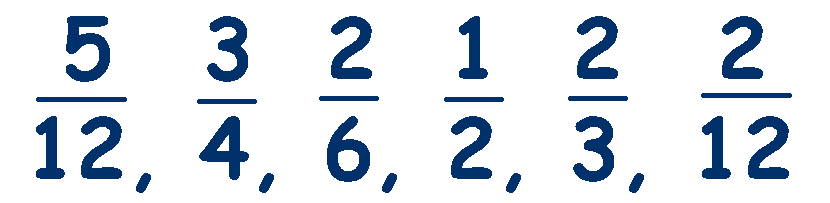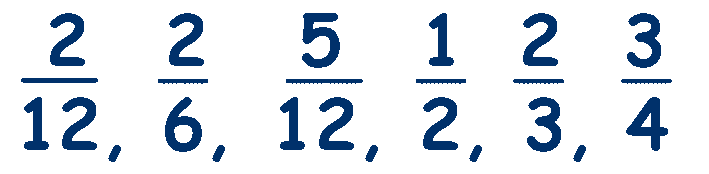# Ordering and Comparing Fractions

Before we start to compare fractions, there are two symbols that we need to explain,

'>' which means 'greater than' and '<' which means 'less than'

### We can only compare fractions if their denominators are the same.

We can easily see that 4/5 is larger than 3/5, because as they both have the same denominator, we are comparing two different amounts of the same thing,
ie. 3 lots of fifths against 4 lots of fifths.

If you are given a set of fractions with the same denominators, such as

## 3/11,  8/11,  1/11,  5/11,  9/11

To put them in order is simply a case of ordering the numerators,

## 1/11,  3/11,  5/11,  8/11,  9/11

If the denominators are different, then you cannot  directly compare them by using the numerators, because they are different amounts of different totals. It's like comparing the size of ½ a grape with ¼ of a grapefruit! And although a ½ is larger than a ¼,
½ of a grape is definitely not larger than ¼ of a grapefruit.

The other thing to notice is that as the numerator increases, the size increases.

## 1/8, 2/8, 3/8, 4/8, 5/8, 6/8

Whereas, if the denominator increases, the size decreases

## 1/10, 1/11, 1/12, 1/13, 1/14, 1/50, 1/100

This is a difficult concept for children to grasp as they are used to a bigger number meaning a bigger size. I usually cut a piece of paper repeatedly into halves to demonstrate that the more pieces there are, the smaller the pieces become.

So, if the denominators are different, we need to make them the same, using our knowledge of equivalent fractions. Look at this example question.

Arrange these fractions in order, smallest first:We need to make all of the denominators the same. What denominator should we choose?

We need to find a denominator that shares the Least Common Multiple.
The smallest multiple that they all share.

The multiples of 2 are: 2, 4, 6, 8, 10, 12, 14, 16 and so on
The multiples of 3 are: 3, 6, 9, 12, 15, 18 and so on
The multiples of 4 are: 4, 8, 12, 16, 20 and so on
The multiples of 6 are: 6, 12, 18, 24 and so on
The multiples of 12 are: 12, 24, 36 and so on

They all share 12, which is the least common multiple, so this needs to be our denominator.

Now, convert all the fractions to equivalent fractions with a denominator of 12. Remember that whatever you do to the bottom, you must do to the top.Now it's just a case of comparing the like fractions - all with a denominator of 12
and writing their equivalent fractions in the correct order.___________________________________________________

Practice comparing and ordering fractions with these resources from Mathsblog

An excellent game from BBC Bitesize on Ordering and Comparing Fractions